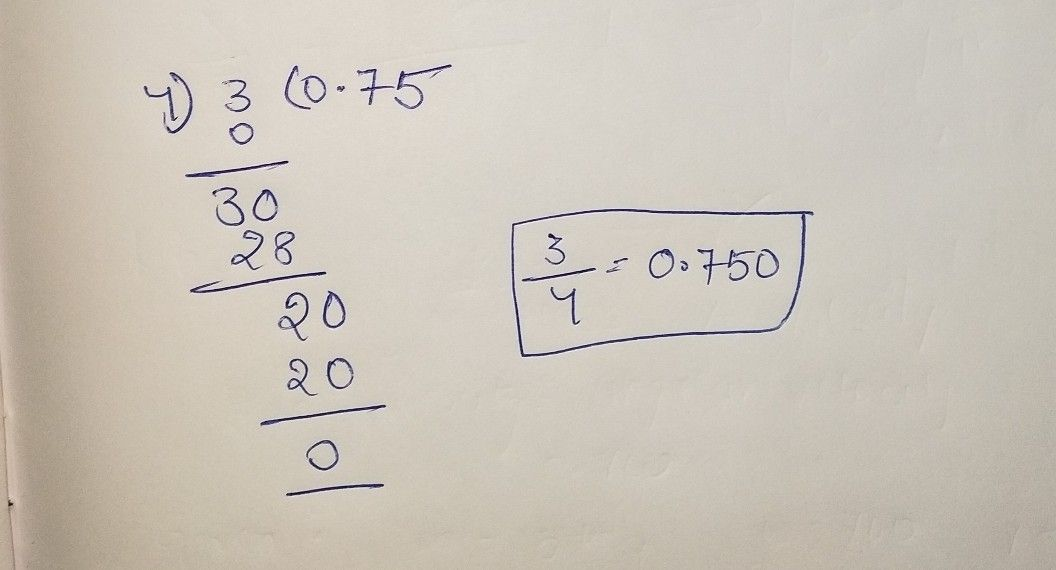Symbol
Problem$6cF$ What's More $A$ A Express each Fraction in Decimal form. Round off your answers into thousandths place. $1$ $\dfrac {3} {4}$
7th-9th grade
Algebra
SolutionQanda teacher - DonMsdStudent
thankyouu